# Determine the electric field strength at 1 km away from a transmitting vertical wire of 1 m length (in free space) in a direction at right angles from the axis of the wire. The wire carries a current of 5 A at a frequency of 1 MHz.

Question
1 views

Determine the electric field strength at 1 km away from a transmitting vertical wire of 1 m length (in free space) in a direction at right angles from the axis of the wire. The wire carries a current of 5 A at a frequency of 1 MHz.

check_circle

Step 1

The rms value of the electric field strength at some distance r away from a transmitting wire can be expressed as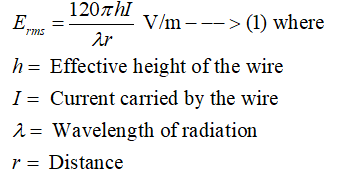Step 2

In this case, the values of the length of the wire, current carried by the wire and the frequency of transmission are given as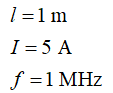Also, the distance at which the electric field is to be measured is given as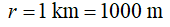Since the electric field is measured at right angles from the axis of the wire, the effective height of the transmitting wire antenna can be expressed as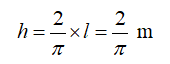Step 3

The wavelength of radiation can be obtained from the frequency of transmission using the following formula: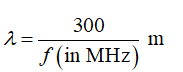Plugging the value of the frequency of transmission into this equation, the value of the wavelength of radiation is found to be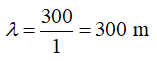...

### Want to see the full answer?

See Solution

#### Want to see this answer and more?

Solutions are written by subject experts who are available 24/7. Questions are typically answered within 1 hour.*

See Solution
*Response times may vary by subject and question.
Tagged in